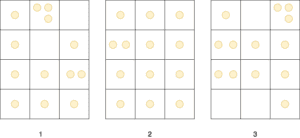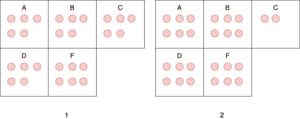## 1. Overview

The pigeonhole principle (also sometimes called the Dirichlet drawer principle) is a simple yet powerful idea in mathematics that can be used to show some surprising things, as we’ll see later. In this article, we’ll first define what the pigeonhole principle is, followed by some examples to illustrate how it can be applied.

Then we’ll discuss the generalized version of the principle and give several examples that use the generalized version. Finally, we’ll show some particularly clever applications that make use of the pigeonhole principle.

## 2. The Basic Pigeonhole Principle

In this section, we’ll first define the basic pigeonhole principle and prove it. Then we’ll show three examples that use this basic principle.

### 2.1. Definition and Proof

The most basic form of the principle refers to pigeons and pigeonholes. Suppose that a set of pigeons fly into a set of pigeonholes. Then if there are more pigeons than pigeonholes, there must be at least one pigeonhole that has more than one pigeon in it.

Shown below are three groups of twelve pigeonholes. Each group has thirteen pigeons (represented by the circles), so there is at least one pigeonhole in each group that has at least two pigeons.We can of course apply this principle to other things besides pigeons and pigeonholes. Formally, we can state the pigeonhole principle like this: If there areboxes andor more objects are placed into these boxes, then there is at least one box that contains two or more of the objects.

Even though the idea seems pretty obvious and almost trivial, we still need to prove that it must be true. The proof is relatively straightforward and goes like this:

Suppose by contradiction that there areboxes andor more objects are placed into these boxes, but there are no boxes with two or more of the objects. This means that every box has at most one object in it.

But if every box has at most one object in it, then there can’t be more thanobjects in the boxes overall. But this is a contradiction since we assumed that there are at leastobjects placed into the boxes!

Next, we give some examples of how the pigeonhole principle can be applied. In each example, we’ll clearly explain what objects correspond to pigeons and what objects correspond to pigeonholes.

### 2.2. First Example

Suppose we have a group of 27 English words. Then there must be at least two words in the group that begin with the same letter. In this case, the 27 English words are the pigeons and we can think of the pigeonholes as buckets that store words that start with a specific letter of the English alphabet.

In this case, there are 26 pigeonholes (one for each letter in the English alphabet) but 27 pigeons, so we can immediately see that there must be at least one bucket with more than one word in it. But a bucket with more than one word implies that there are at least two words that start with the same letter.

### 2.3. Second Example

In any group of 367 people, there must be at least two people with the same birthday. Here we can think of a person as a pigeon and a room that has all the people who have a specific birthday as a pigeonhole. Since there are only 366 possible birthdays, we have 366 rooms.

But if we put 367 people in 366 rooms, then we know that there must be at least one room with at least two people in it. This means that there must be at least two people with the same birthday.

### 2.4. Third Example

Let’s say we know the maximum number of hairs that a person can have on his head. Letbe this number. Then in any group of more thanpeople, there must be at least two people with the same number of hairs on their heads.

A person represents a pigeon and a room with people that have a specific number of hairs on their heads represents a pigeonhole. Since we have more people than rooms, there must be at least one room with at least two people in it.

Now the average number of hairs on a person’s head is about 100,000, so this means that the maximum is probably at most a few hundred thousand. This means that in any group of say 300,000 people, it’s guaranteed that there are at least two people with the same number of hairs on their heads!

## 3. The Generalized Pigeonhole Principle

Here we’ll define and prove the generalized version of the pigeonhole principle. Then we’ll see three examples that use this generalized version.

### 3.1. Definition and Proof

We can say even more when the number of objects is more than a multiple of the number of boxes. For example, in any set of 21 decimal digits, there must be 3 that are the same. This is because if 21 objects are put into 10 boxes, there must be at least one box with at least 3 objects in it.

We can formally express this notion as the generalized pigeonhole principle. The generalized pigeonhole principle states that ifobjects are placed inboxes, then there must be at least one box with at leastobjects in it. Now we’ll prove this statement.

Suppose thatobjects are placed intoboxes, but every box contains at mostobjects. Then the total number of objects is at most, but this value is less than. This is because we know that, so if substitute the expressionin the place ofin the expressionthen we’ll getwhich must be bigger in value.

Thus, we get a contradiction since there areobjects in total.

Next, we’ll give some examples of how we can apply the generalized pigeonhole principle. In each example, we’ll clearly explain what corresponds to objects and what corresponds to boxes.

### 3.2. First Example

In any group of 100 people there is at leastwho were born in the same month. Here the objects we are placing are the people and the boxes can be imagined as rooms that have people who were born in a specific month. Since we have 100 people and 12 rooms (one for each month of the year), there must be a room with at least 9 people in it.

### 3.3. Second Example

To guarantee that at least three cards of the same suit are chosen, we must select at least nine cards from a standard deck of 52 cards. In this case, we can think of the boxes as marked with a particular suit, and as we select cards from the deck we place them in the box with the same suit as the selected card. The generalized pigeonhole principle tells us that ifcards are selected, there is at least one box containing at leastcards.

So ifthen we know that at least three cards of the same suit must’ve been selected. The smallest possible value thatcan take for this condition to be true is 9, so that at least nine cards must be selected.

### 3.4. Third Example

The minimum number of students needed in a class to ensure that at least six students receive the same grade is 26 if there are five possible grades (e.g. A, B, C, D, and F). We can think of each object as a person and each box as a room with people that have a specific grade. The minimum number of students needed to satisfy the condition is equal to the smallest integersuch that, and the smallest such integeris 26.

Shown below are two instances of this third example. In both cases, we have 5 boxes that represent the five possible grades and 26 red circles representing 26 students that are assigned some grade. In both cases, there is at least one box containing at least six red circles which means that there are at least six students who got the same grade.## 4. Clever Applications of the Pigeonhole Principle

In this section, we’ll discuss a couple of interesting applications of the pigeonhole principle that require a little bit more sophistication.

### 4.1. First Example

Among anyintegers such that each integer is at most, there must be an integer that divides one of the other integers. We can write each of theintegersas a power of 2 times an odd integer. So letfor, whereis a nonnegative integer andis odd. The integersare all odd positive integers less than.

It follows from the pigeonhole principle that two of the integersmust be equal, since there are onlyodd positive integers less than. So there must exist integersandsuch that. Letbe the common value ofand.

Then we have thatand. Therefore, ifthendivides, whereas if, thendivides.

### 4.2. Second Example

Suppose that there is a sports team that plays at least one game a day for 30 days, but no more than 45 games in total. Then there must be a period of some consecutive days during which the team must play exactly 14 games. We’ll now prove this statement.

Letbe the number of games played on or before theth day of the 30 day period. Thenis an increasing sequence of distinct positive integers, with. Furthermore,is also an increasing sequence of distinct positive integers, with.

The 60 positive integersare all less than or equal to 59. The pigeonhole principle then tells us that two of these integers are equal. Since the integersare all distinct and the integersare also all distinct, there must be indicesandwith.

But this means that exactly 14 games were played from dayto day.

## 5. Conclusion

In this article, we explored the pigeonhole principle through some definitions and examples.

First, we discussed the most basic form of the pigeonhole principle along with its proof. Then we gave some examples that make use of this basic principle.

Then we introduced the generalized version of the pigeonhole principle and proved it as well. We also saw some examples that make use of this generalized version.

Finally, we saw some more interesting applications of the pigeonhole principle. These final examples show that with a little bit more sophistication combined with the pigeonhole principle, we can prove some pretty impressive results.